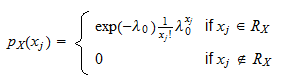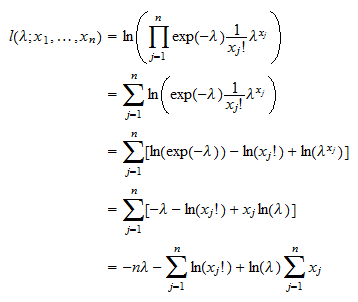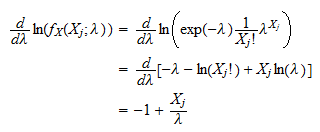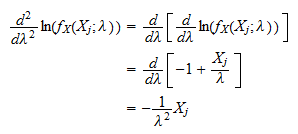StatLect

# Poisson distribution - Maximum Likelihood Estimation

This lecture explains how to derive the maximum likelihood estimator (MLE) of the parameter of a Poisson distribution. Before reading this lecture, you might want to revise the lectures about maximum likelihood estimation and about the Poisson distribution.## Assumptions

We assume to observeinependent draws from a Poisson distribution. In more formal terms, we observe the firstterms of an IID sequenceof Poisson random variables. Thus, the probability mass function of a term of the sequenceiswhereis the support of the distribution andis the parameter of interest (for which we want to derive the MLE). Remember that the support of the Poisson distribution is the set of non-negative integer numbers:To keep things simple, we do not show, but we rather assume that the regularity conditions needed for the consistency and asymptotic normality of the maximum likelihood estimator ofare satisfied.

## The likelihood function

The likelihood function isProof

Theobservations are independent. As a consequence, the likelihood function is equal to the product of their probability mass functions:Furthermore, the observed valuesnecessarily belong to the support. So, we have## The log-likelihood function

The log-likelihood function isProof

By taking the natural logarithm of the likelihood function derived above, we get the log-likelihood:## The maximum likelihood estimator

The maximum likelihood estimator ofisProof

The MLE is the solution of the following maximization problemThe first order condition for a maximum isThe first derivative of the log-likelihood with respect to the parameterisImpose that the first derivative be equal to zero, and getTherefore, the estimatoris just the sample mean of theobservations in the sample. This makes intuitive sense because the expected value of a Poisson random variable is equal to its parameter, and the sample mean is an unbiased estimator of the expected value.

## Asymptotic variance

The estimatoris asymptotically normal with asymptotic mean equal toand asymptotic variance equal toProof

The score isThe Hessian isThe information equality implies thatwhere we have used the fact that the expected value of a Poisson random variable with parameteris equal to. Finally, the asymptotic variance isThus, the distribution of the maximum likelihood estimatorcan be approximated by a normal distribution with meanand variance.

The book

Most of the learning materials found on this website are now available in a traditional textbook format.

Glossary entries
Share